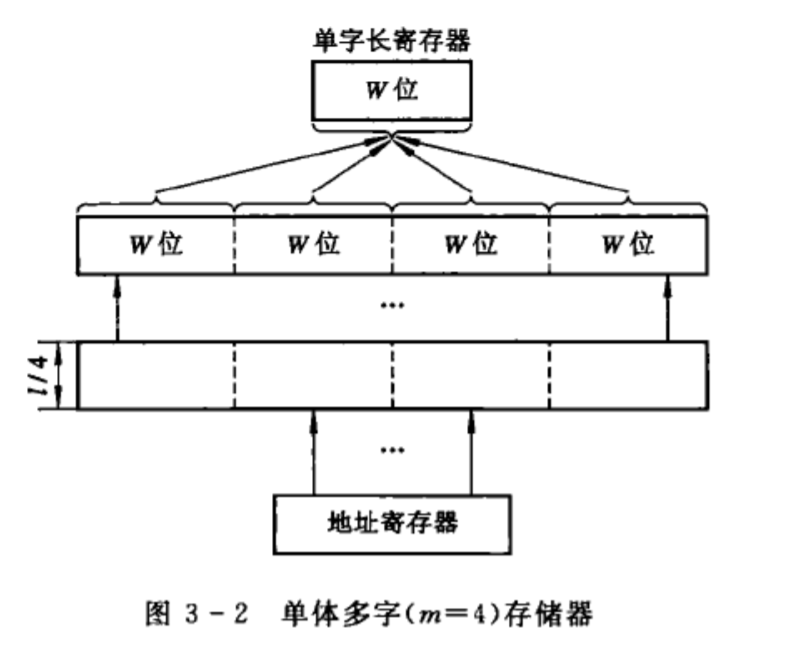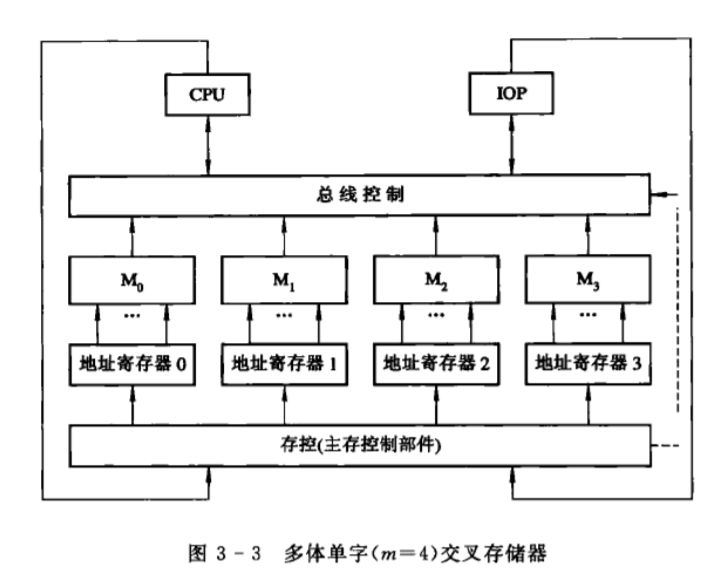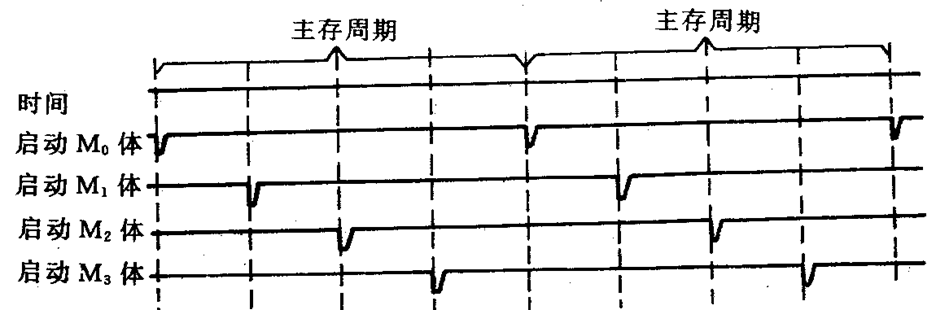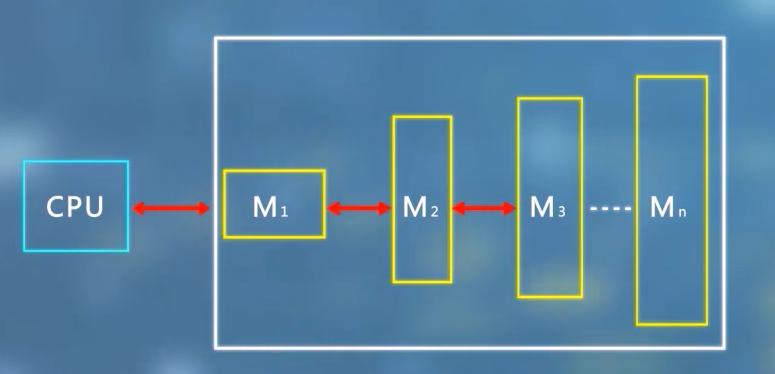﻿ 计算机存储体系：量化分析与并行存储

1. 局部性原理
2. 加快经常性事件

1. 容量(S)

(w):存储器字长

(l):字数

(m):并行工作存储体的个数

1. 速度（访问时间）

## 并行存储系统

### 单体多字存储器• 如果一次读取的m个指令字中有分支跳转指令，而且成功了，意味着该指令后面的指令是无用的。

• 这一次取到的m个数据不一定都是有用的，假设当前的指令需要多个操作数，也不一定正好全部一次取到。

• 假设这个存储周期存在对同一个存储单元的写和读，写入之后那读的操作就发生错误了。

### 多体单字存储器• 高位交叉编址

• 低位交叉编址

### 分时启动

m个存储体分时启动，实际上是一种采用流水线方式工作的并行存储器。1)模m越高，存贮器数据总线越长，导致传输延迟增加；
2)系统效率问题，对于顺序取指，效率可以提高m倍，但遇到转移指令，效率就会下降。

## 存储系统的量化分析

### 多级层次结构(S_1<S_2<S_3<S_n)

(M_1>M_2>M_3>M_4)

### 存储系统的性能参数• 每位价格(C)

(C=frac{C_1S_1+C_2S_2}{S_1+S_2})

(S_1<<S_2),(C)约等于(C_2)

• 命中率(H)与不命中率(F)

(N_1)代表在(M_1)找到信息的次数

(H=frac{N_1}{N_1+N_2})

(F=1-H)

• 等效访问时间

• 命中(T_1)：访问时间为(T_1)，命中的概率为(H)
• 不命中(T_M)：从(M_2)发出访问请求到整个数据块装入(M_1)所需要的时间：(T_2+T_B)

(T_B)是传送一个信息块的时间。

[T_A = HT1+(1-H)(T_1+T_M)=T_1+(1-H)T_M ]

[T_A = T_1+FT_M ]

[e=frac{T_1}{T_A}=frac{HT_1}{HT_1+(1-H)T_M}=frac{1}{H+(1-H)r} ]

(r)是指相邻两级的访问时间比(frac{T1}{T_2}),不考虑传送时间的情况下。

• 减小相邻两级的访问速度差距

• 减小相邻两级存贮器的容量差

## 来源

 计算机系统结构课本

 计算机系统结构 华中科技大学 中国大学MOOC